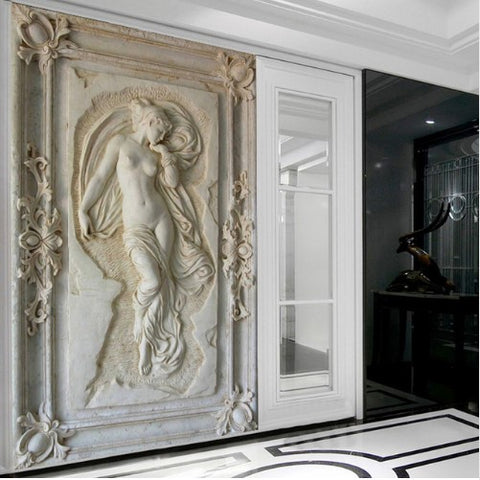## Elegant Classic Art Nude Female Design Wall Mural 3D Relief Style Wallpaper

• \$39.95

IMPORTANT: PLEASE READ! \$39.95 is for  (1 square meter) 1 piece. only.  It is very small, about 4'7" (H) x 2'4" (W). Do NOT order 1 piece (1 square meter) if you want to cover a complete door or feature area.  You will need more.  The example pictures show a feature wall covered with wallpaper. You need to measure your wall.  Please see below product details how to calculate how many pieces you need.

Product Details

• Function: Waterproof, Sound-Absorbing, Heat Insulation, Smoke-Proof, Moisture-Proof, Mold-Proof, Soundproof, Anti-static
• Feature: Formaldehyde-free, Mildew Resistant, Extra Thick,Environment Friendly
• Textile Wallpaper Material: Non-Woven, Wood Fiber

How to calculate how many pieces you need:

1). Measure your wall.  Convert your measurements to centimeters (you can use any online metric converter).
2). If your wall is 360cm width x 240cm height for example, multiply those numbers: 360 x 240 = 8.6.  So you would need to buy 9 pieces.

1. Click "add to cart". It will take you to checkout.

2. Enter the number you wish to purchase. Total will be calculated for you.

Here are some fixed sizes if you prefer to choose one of these:

Quantity 1  :  1 square meter = 140cm(H) x 70cm(W) (4'7" x 2'4") = \$39.95

Quantity 2  :  2 square meter = 200cm(H) x 100cm(W) (6'7" x 3'3") = \$79.90

Quantity 3  :  3 square meter = 220cm(H) x 140cm(W) (7'3" x 4'7") = \$119.85

Quantity 4  :  4 square meter = 250cm(H) x 160cm(W) (8'2" x 5'3") = \$159.80

Quantity 5  :  5 square meter = 280cm(H) x 180cm(W) (9'2" x 5'11") = \$199.75

Quantity 6  :  6 square meter = 300cm(H) x 200cm(W) (9'10" x 6'7") = \$239.70

Quantity 7  :  7 square meter = 330cm(H) x 210cm(W) (10'10" x 6'11") = \$279.65

Quantity 8  :  8 square meter = 360cm(H) x 230cm(W) (11'10" x 7'6") = \$319.60

Quantity 9  :  9 square meter = 380cm(H) x 240cm(W) (12'5" x 7'10") = \$359.55

Quantity 10 :  10 square meter = 400cm(H) x 250cm(W) (13'1" x 8'2") = \$399.50

Quantity 11 : 11 square meter = 420cm(H) x 260cm(W) (13'9'' x 8'6'') =  \$439.45

Quantity 12 : 12 square meter = 440cm(H) x 270cm(W) (14'5" x 8'10") = \$479.40

Quantity 13 : 13 square meter = 460cm(H) x 280cm(W) (15'1'' x 9'2'') = \$519.35

Quantity 14 : 14 square meter = 480cm(H) x 290cm(W) (15'9'' x 9'6'') =  \$559.30

Quantity 15 : 15 square meter= 500cm(H) x 300cm(W) (16'5'' x 9'10'') = \$599.25

Quantity 16 : 16 square meter=500cm(H) x 320cm(W) (16'5" x 10'6") = \$639.20

We Also Recommend